## Solution to 1986 Problem 4

 (A) The amplitude is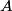$A$ not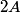$2A$. (B) The direction of the velocity can be found by required that the argument of the sine function is a constant: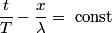\begin{align*}\frac{t}{T} - \frac{x}{\lambda} = \mbox{ const}\end{align*} Increasing t a little bit requires you to increase x a little bit as well in order to maintain this equality, so the velocity in the positive x-direction. (C) The period is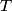$T$. (D)$x$ and$t$ are variables here, so this cannot be right. (E) Consider the equation above. Let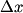$\Delta x$ be the change in$x$ during a small time interval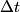$\Delta t$. Then, in order to maintain that equality we must have that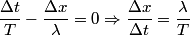\begin{align*}\frac{\Delta t}{T} - \frac{\Delta x}{\lambda} = 0 \Rightarrow \frac{\Delta x}{\Delta t} = \frac{ \lambda}{T}\en...So, answer (E) is correct.